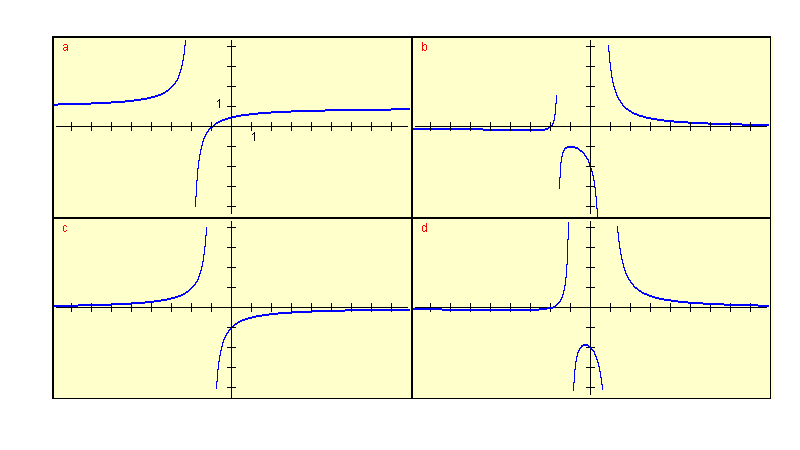# Graphs of Question 5

 Question 5: Identify the possible graph of the rational function f given by f(x) = (x + 2) / (x 2 -1)Other pages in this website related to solving the questions in this self test can be found at

Home Page - Online Calculators - Trigonometry - Antennas - Graphing - Precalculus Tutorials - Calculus Tutorials
Calculus Questions - Geometry Tutorials - Precalculus Applets - Applied Math - Precalculus Questions and Problems -
Equations, Systems and Inequalities - Geometry Calculators - Math Software - Elementary Statistics -
Author - e-mail

Updated: 8 November 2008 (A Dendane)